### ASM to Sega Genesis Platform

Would you like to react to this message? Create an account in a few clicks or log in to continue.
ASM to Sega Genesis Platform

All about assembly programming in the Sega Genesis console.

+4
Green Snake
i_<3_knucles
calebjhuhlu
OuricoDoido
8 posters

# Homing Attack in Sonic 1 - Step 1 - Creating the Homing Attack, and make it workOuricoDoido

Mensagens : 46
Data de inscrição : 2011-01-09
Current Project : Sonic Open Source Project
Creating the Homing Attack

To add this skill to Sonic, you need go to Obj01_MdJump2 in sonic1.asm, and add this line:
Code:
`      bsr.w   Sonic_Homingattack`
Code:
`Obj01_MdJump2:            ; XREF: Obj01_Modes      bsr.w   Sonic_Homingattack      bsr.w   Sonic_JumpHeight      bsr.w   Sonic_ChgJumpDir      bsr.w   Sonic_LevelBound      jsr   ObjectFall      btst   #6,\$22(a0)      beq.s   loc_12EA6      subi.w   #\$28,\$12(a0)`

Go below Sonic_Roll subroutine (below this text: ; End of function Sonic_Roll) , and Add this (a normal skill code):
Code:
`; ---------------------------------------------------------------------------; Subroutine Sonic_Homingattack; ---------------------------------------------------------------------------; ||||||||||||||| S U B   R O U T   I N E |||||||||||||||||||||||||||||||||||||||Sonic_Homingattack:      move.b   (\$FFFFF603).w,d1   ; read controller      andi.b   #\$40,d1            ; is A pressed?      beq.w   Sonic_HA_rts      ; if not, branchSonic_HA_rts:      rts                  ; return; Command of Homingattack end here`
If you want to "hold A" instead of "press A", modify \$FFFFF603 to \$FFFFF604.

For other buttons, change the #\$40 to:
Code:
`A = #\$40B = #\$10C = #\$20Start = #\$80Up = #1Down = #2Left = #4Right = #8`

Doing the Homing Attack work

Now, we start with main homing attack command, to move the Sonic in enemy direction.

Between these lines:
Code:
`      beq.w   Sonic_HA_rts      ; if not, branch----->>>>>>        HERE        <<<<<<-----      Sonic_HA_rts:`

Code:
`      lea      (\$FFFFD800).w,a1   ; start at the first level object RAM; ---------------------------------------------------------------------------HA_enemylist:      tst.b   (a1)            ; is a Null object      bne.s   HA_calcdistance      ; if not, branchHA_nextobject:      adda.w  #\$40,a1         ; jump to next object RAM entry      cmpa.w  #\$F000,a1      ; already tested all object RAM entry?      blt.s  HA_enemylist   ; if not, return to enemy list      rts; ---------------------------------------------------------------------------HA_calcdistance:   ; distance calculator      move.w  8(a1),d1   ; move the object x-position to d1      move.w  \$C(a1),d2   ; move the object y-position to d2      sub.w  8(a0),d1   ; sub sonic x-position of object x-position      sub.w  \$C(a0),d2   ; sub sonic y-position of object y-position; ---------------------------------------------------------------------------HA_Move:      jsr      (CalcAngle).l   ; calculates the angle      jsr      (CalcSine).l   ; calculates the sine and the cosine      muls.w  #\$C,d1         ; multiply cosine by \$C      move.w  d1,\$10(a0)      ; move d1 to X-velocity      muls.w  #\$C,d0         ; multiply sine by \$C      move.w  d0,\$12(a0)      ; move d0 to Y-velocity`
Compile and test, jump and hold or press A to you see what happens. (Note: If you used the "Hold A" instruction, you will see better the effect.)
In this code, the Sonic is going vs any object (rocks, bridge, spikes, .....)

I will explain this code by parts (the part 3, 4 and 5 have already been explained in Step 0.)

Part 1:
Code:
`      lea      (\$FFFFD800).w,a1   ; start at the first level object RAM`
This code aims to set the address \$FFFFD800 in a1;

Because in Sonic 1, \$FFFFD800 address, where is located "the first level object".
This code is completed by the 2nd part, to understand this part, read the 2nd part.

Part 2:
Code:
`   HA_enemylist:         tst.b   (a1)            ; is a Null object         bne.s   HA_calcdistance      ; if not, branch   HA_nextobject:         adda.w  #\$40,a1         ; jump to next object RAM entry         cmpa.w  #\$F000,a1      ; already tested all object RAM entry?         blt.s  HA_enemylist   ; if not, return to enemy list         rts`
This code aims to locate any existing object.

Here is the difference between the basic Homing Attack and the Pinball Bumper, in Pinball Bumper the two objects already have determined (the Sonic and own Pinball Bumper as you saw in Step 0),we already know the Pinball Bumper's RAM address (since the calculation is done within the object's subroutines, a0 being the address) and the Sonic's address in RAM is always fixed (\$FFFFD000), no needing find the Sonic in RAM.

But in the Homing Attack, Sonic can attack any object, which in fact is not fixed in RAM, according the objects are being erased in the RAM (when the Sonic destroy him for example), new objects that will be loaded, will earn a RAM position, which may be or not, of the deleted object.

Knowing that the positions of objects in RAM are not fixed, we will be forced to find them in RAM, this way the Homing Attack is not limited to only one object.

Code:
`         tst.b   (a1)            ; is a Null object         bne.s   HA_calcdistance      ; if not, branch`
This code checks if the object exists, if it does not exist, the subroutine continues to read, searching for another object (in the code below),
but if it exists, go to HA_calcdistance subroutine to calculate the distance, angle, sine and cosine .... and execute the Homing Attack.

Code:
`         adda.w   #\$40,a1         ; jump to next object RAM entry         cmpa.w   #\$F000,a1      ; already tested all object RAM entry?         blt.s   HA_enemylist   ; if not, return to enemy list         rts`
If the object does not exist or is not part of the list of enemies (as we shall see in the step 2), will run this code, which aims to jump to the next object in RAM.

Now an explanation line by line:
1st - each object has a size of \$40 bytes, so "adda.w #\$40, a1", to skip \$40 bytes in a1, going to the next object.
2nd - "level object variable space" ends at address \$FFFFF000, so "cmpa.w #\$F000, a1", compares a1 with address where ends "level object variable space".
3rd - The "blt.s HA_enemylist" is if the RAM position that is in a1 is less than the address where ends "level object variable space", keep searching for an object, returning to the enemy list.

Part 3:
Code:
`      move.w  8(a1),d1   ; move the object x-position to d1      move.w  \$C(a1),d2   ; move the object y-position to d2      sub.w  8(a0),d1   ; sub sonic x-position of object x-position      sub.w  \$C(a0),d2   ; sub sonic y-position of object y-position`
This is the calculation to obtain the object's coordinates as if Sonic's coordinates is (x=0, y=0), so we can calculate the angle, as explained in Step 0.

Part 4:
Code:
`      jsr      (CalcAngle).l   ; calculates the angle      jsr      (CalcSine).l   ; calculates the sine and the cosine`
Here is done the math to figure out the angle (based on information received from Part 3), and figure out the Sine and Cosine (based on the angle).

Part 5:
Code:
`      muls.w  #\$C,d1         ; multiply cosine by \$C      move.w  d1,\$10(a0)      ; move d1 to X-velocity      muls.w  #\$C,d0         ; multiply sine by \$C      move.w  d0,\$12(a0)      ; move d0 to Y-velocity`

This calculation serves to increase the sine and cosine's value that are very low, as explained in Step 0.
And after increase, set the sine at Sonic's y-velocity and set the cosine at Sonic's x-velocity.calebjhuhlu

Mensagens : 55
Data de inscrição : 2011-06-16
Also, When Applying What Button to press, Modify \$40 to \$70 if you want to use homing attack on A, B, And C.
EDIT: Change it to 50 if you want it to be A and B.
Change it to 60 if you want it A and C
And For B and C it would be 30.
Just thought I'd Share.Last edited by calebjhuhlu on Fri Jun 24, 2011 6:09 am; edited 1 time in totali_<3_knucles

Mensagens : 19
Data de inscrição : 2011-06-20
lul tweeker told me u stole his code he r pissedGreen Snake

Mensagens : 2185
Data de inscrição : 2012-04-07
Localização : I do not even know
Current Project : nopeGreen Snake

Mensagens : 2185
Data de inscrição : 2012-04-07
Localização : I do not even know
Current Project : nopehacker___

Mensagens : 1974
Data de inscrição : 2013-05-06
Localização : 41.383863, 108.866009
Current Project : Breathing nitrogen

oGreen Snake

Mensagens : 2185
Data de inscrição : 2012-04-07
Localização : I do not even know
Current Project : nope
hacker___ wrote:
oThomasSpeedrunner

Mensagens : 177
Data de inscrição : 2013-07-31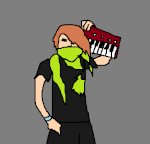SonicVaan

Mensagens : 100511
Data de inscrição : 2013-07-25
Localização : ASMTSG
Current Project : BEING THE KING OF THE FORUM
OuricoDoido wrote:Creating the Homing Attack

To add this skill to Sonic, you need go to Obj01_MdJump2 in sonic1.asm, and add this line:
Code:
` bsr.w Sonic_Homingattack`
Code:
`Obj01_MdJump2: ; XREF: Obj01_Modes bsr.w Sonic_Homingattack bsr.w Sonic_JumpHeight bsr.w Sonic_ChgJumpDir bsr.w Sonic_LevelBound jsr ObjectFall btst #6,\$22(a0) beq.s loc_12EA6 subi.w #\$28,\$12(a0)`
Go below Sonic_Roll subroutine (below this text: ; End of function Sonic_Roll) , and Add this (a normal skill code):
Code:
`; ---------------------------------------------------------------------------; Subroutine Sonic_Homingattack; ---------------------------------------------------------------------------; ||||||||||||||| S U B R O U T I N E |||||||||||||||||||||||||||||||||||||||Sonic_Homingattack: move.b (\$FFFFF603).w,d1 ; read controller andi.b #\$40,d1 ; is A pressed? beq.w Sonic_HA_rts ; if not, branchSonic_HA_rts: rts ; return; Command of Homingattack end here`
If you want to "hold A" instead of "press A", modify \$FFFFF603 to \$FFFFF604.

For other buttons, change the #\$40 to:
Code:
`A = #\$40B = #\$10C = #\$20Start = #\$80Up = #1Down = #2Left = #4Right = #8`

Doing the Homing Attack work

Now, we start with main homing attack command, to move the Sonic in enemy direction.

Between these lines:
Code:
` beq.w Sonic_HA_rts ; if not, branch----->>>>>>   HERE   <<<<<<----- Sonic_HA_rts:`
Code:
` lea (\$FFFFD800).w,a1 ; start at the first level object RAM; ---------------------------------------------------------------------------HA_enemylist: tst.b (a1) ; is a Null object bne.s HA_calcdistance ; if not, branchHA_nextobject: adda.w  #\$40,a1 ; jump to next object RAM entry cmpa.w  #\$F000,a1 ; already tested all object RAM entry? blt.s   HA_enemylist ; if not, return to enemy list rts; ---------------------------------------------------------------------------HA_calcdistance: ; distance calculator move.w  8(a1),d1 ; move the object x-position to d1 move.w  \$C(a1),d2 ; move the object y-position to d2 sub.w   8(a0),d1 ; sub sonic x-position of object x-position sub.w   \$C(a0),d2 ; sub sonic y-position of object y-position; ---------------------------------------------------------------------------HA_Move: jsr (CalcAngle).l ; calculates the angle jsr (CalcSine).l ; calculates the sine and the cosine muls.w  #\$C,d1 ; multiply cosine by \$C move.w  d1,\$10(a0) ; move d1 to X-velocity muls.w  #\$C,d0 ; multiply sine by \$C move.w  d0,\$12(a0) ; move d0 to Y-velocity`
Compile and test, jump and hold or press A to you see what happens. (Note: If you used the "Hold A" instruction, you will see better the effect.)
In this code, the Sonic is going vs any object (rocks, bridge, spikes, .....)

I will explain this code by parts (the part 3, 4 and 5 have already been explained in Step 0.)

Part 1:
Code:
` lea (\$FFFFD800).w,a1 ; start at the first level object RAM`
This code aims to set the address \$FFFFD800 in a1;

Because in Sonic 1, \$FFFFD800 address, where is located "the first level object".
This code is completed by the 2nd part, to understand this part, read the 2nd part.

Part 2:
Code:
` HA_enemylist: tst.b (a1) ; is a Null object bne.s HA_calcdistance ; if not, branch HA_nextobject: adda.w  #\$40,a1 ; jump to next object RAM entry cmpa.w  #\$F000,a1 ; already tested all object RAM entry? blt.s   HA_enemylist ; if not, return to enemy list rts`
This code aims to locate any existing object.

Here is the difference between the basic Homing Attack and the Pinball Bumper, in Pinball Bumper the two objects already have determined (the Sonic and own Pinball Bumper as you saw in Step 0),we already know the Pinball Bumper's RAM address (since the calculation is done within the object's subroutines, a0 being the address) and the Sonic's address in RAM is always fixed (\$FFFFD000), no needing find the Sonic in RAM.

But in the Homing Attack, Sonic can attack any object, which in fact is not fixed in RAM, according the objects are being erased in the RAM (when the Sonic destroy him for example), new objects that will be loaded, will earn a RAM position, which may be or not, of the deleted object.

Knowing that the positions of objects in RAM are not fixed, we will be forced to find them in RAM, this way the Homing Attack is not limited to only one object.

Code:
` tst.b (a1) ; is a Null object bne.s HA_calcdistance ; if not, branch`
This code checks if the object exists, if it does not exist, the subroutine continues to read, searching for another object (in the code below),
but if it exists, go to HA_calcdistance subroutine to calculate the distance, angle, sine and cosine .... and execute the Homing Attack.

Code:
` adda.w #\$40,a1 ; jump to next object RAM entry cmpa.w #\$F000,a1 ; already tested all object RAM entry? blt.s HA_enemylist ; if not, return to enemy list rts`
If the object does not exist or is not part of the list of enemies (as we shall see in the step 2), will run this code, which aims to jump to the next object in RAM.

Now an explanation line by line:
1st - each object has a size of \$40 bytes, so "adda.w  #\$40, a1", to skip \$40 bytes in a1, going to the next object.
2nd - "level object variable space" ends at address \$FFFFF000, so "cmpa.w  #\$F000, a1", compares a1 with address where ends "level object variable space".
3rd - The "blt.s  HA_enemylist" is if the RAM position that is in a1 is less than the address where ends "level object variable space", keep searching for an object, returning to the enemy list.

Part 3:
Code:
` move.w  8(a1),d1 ; move the object x-position to d1 move.w  \$C(a1),d2 ; move the object y-position to d2 sub.w   8(a0),d1 ; sub sonic x-position of object x-position sub.w   \$C(a0),d2 ; sub sonic y-position of object y-position`
This is the calculation to obtain the object's coordinates as if Sonic's coordinates is (x=0, y=0), so we can calculate the angle, as explained in Step 0.

Part 4:
Code:
` jsr (CalcAngle).l ; calculates the angle jsr (CalcSine).l ; calculates the sine and the cosine`
Here is done the math to figure out the angle (based on information received from Part 3), and figure out the Sine and Cosine (based on the angle).

Part 5:
Code:
` muls.w  #\$C,d1 ; multiply cosine by \$C move.w  d1,\$10(a0) ; move d1 to X-velocity muls.w  #\$C,d0 ; multiply sine by \$C move.w  d0,\$12(a0) ; move d0 to Y-velocity`
This calculation serves to increase the sine and cosine's value that are very low, as explained in Step 0.
And after increase, set the sine at Sonic's y-velocity and set the cosine at Sonic's x-velocity.JoshDP

Mensagens : 1682
Data de inscrição : 2014-10-05
Just an annual year bump!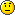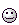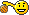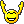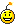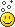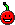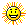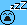Green Snake

Mensagens : 2185
Data de inscrição : 2012-04-07
Localização : I do not even know
Current Project : nope